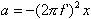This is the defining equation for simple harmonic motion. The factor (2 pi f)^2 is a positive constant.   The equation states that the acceleration of an object is equal to the negative of that constant times the position of the object.  The constant is the square of 2 pi times the frequency of oscillations of the object.   Hence anytime you can use Newton's second law to deduce that the acceleration of an object is equal to the negative of a constant times the position of the object, you know that the object undergoes simple harmonic motion, and, that the constant is equal to (2 pi f)^2 where f is the frequency at which the object oscillates.

Note: The equation at the top of this page is for the case of an object undergoing linear motion.   The corresponding rotational motion equation:  alpha = - (2 pi f)^2    theta
also holds.